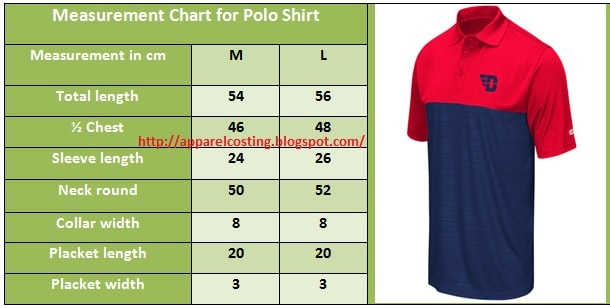# Apparel Costing

## How to Calculate Apparel Costing for Polo Shirt?

Knitted Garments Costing and Consumption in Apparel Sector:
Costing and consumption or apparel export order is the main task for the apparel merchandisers. Both works are not too much tough if apparel merchandiser follows the actual method for those. In another article I have shared an article about How to calculate apparel costing for T-Shirt, but now I have presented here the actual fabric consumption and apparel costing method for knitted polo shirt.

Polo Shirt Costing and Consumption Method in Apparel Industry:
Find out the price of a Polo T-shirt by following the given measurement chart and apparel specifications:Fig: Polo shirt costing method in apparel industry
 Polo Shirt Specifications Style of  Polo Shirt Polo T-shirt with flat knit collar Fabric Body - 95% Cotton single jersey and 5% Lycra Collar – (2×2) Rib, 100% cotton G.S.M 180 G.S.M for body fabric and 220 for collar Color Light green Print Print at chest Size Ratio S:M:L:XL = (1:1.5:2:0.5) Quantity of Order 20000 pcs

Solution:
Fabric Consumption Calculation or Apparel Weight Calculation:

To calculate fabric consumption for polo shirt, we will apply the following formula:

Apparel weight calculation or fabric consumption (Single jersey fabric),

[{T.L + S.L + (2× H.A) + (2 × S.A)} × (1/2 Chest + S.A)] × G.S.M × 2
………………………………………………………………………..........…          … (A)
10000

Again,

Flat knit collar weight calculation (Rib fabric),

{Neck round × (Collar width + S.A) × G.S.M}
……………………………………………………          ……….. (B)
10000

N.B: Flat knit collar is single layer fabric. So, no need to multiply by 2.

Again,

Flat placket weight calculation (Single jersey fabric),

{(Placket length + S.A) × (Placket width + S.A) × G.S.M × 3}
………………………………………………………….....………          …….. (C)
10000

N.B: For placket, there is 3 or 4 fold. So, it is multiplied by 3 or 4.

Where,

T.L is stands for Total length,
S.A stands for Seam allowance,
H.A stands for Hem allowance,
S.L stands for Sleeve length.

Fabric consumption calculation for middle size i.e. L Size:

Let,
Seam allowance =2cm,
Hem allowance = 2cm,

So,
From equation-A, we get,

[{56+26+ (2×2) + (2×2)} × (48+2)] ×180×2
……………………………………………………….
10000

= 162gms
= 0.162kg

Again,
From equation-B, we get,

{(52 × (8+2) ×220}
……………………………..
10000

= 11.44gms
= 0.012kg

Again,
From equation- (C), we get,

{(20+2) × (3+2) × 180 × 3}
…………………………………..
10000

= 5.94gms
= 0.006kg

So,
L size fabric consumption (for single jersey) is,
= (0.162+0.006) kg
= 0.168kg

Again,
L size fabric consumption (for 2×2 rib fabric) is 0.012kg

Calculation of Fabric Cost Per Kg:
 Parameters Costing for S/J Costing for Lycra Yarn price per kg Cotton 95% = Rs. 240×95%= Rs. 228 Rs. 268.00 Rs. 190.00 Lycra 5% = Rs. 800×5% = Rs. 40 Knitting rate per kg (S/J Lycra) Rs. 15.00 Rs. 14.00 Dyeing rate per kg Rs. 60.00 Rs. 60.00 Printing rate per kg Rs. 0.00 Rs. 0.00 Compacting rate per kg Rs. 6.00 Rs. 6.00 Heat setting rate per kg (Synthetic fabric) Rs. 6.00 Rs. 0.00 Total Rs. 355.00 Rs. 270.00 Process loss (10%) (+) Rs. 35.50 Rs. 27.00 Fabric cost per kg Rs. 390.50 Rs. 297.00

Cost of Fabric Calculation per Pc Apparel:

Cost of fabric per apparel,
= (Apparel weight or fabric consumption × Fabric cost per kg)

For single jersey fabric,
= 0.168kg × Rs. 390.50
= Rs. 65.60

You may follow Top 20 Knit Garment Factory in Bangladesh

For (2×2) Rib fabric,
= 0.012kg × Rs. 297.00
= Rs. 3.56

Total fabric cost stands at,
= Rs. 65.60 + Rs. 3.56
= Rs. 69.16

Apparel Costing Format for Knitted Polo Shirt:
The cost of the knitted polo shirt is calculated as in the below:
 SL No. Particulars Costing 01 Cost of fabric per pc apparel Rs. 67.30 02 Cost of making cost Rs. 15.00 03 Chest printing Rs. 8.00 Trimmings and accessories Main label Rs. 0.60 Rs. 5.55 Wash care label Rs. 0.30 Button Rs. 1.00 Pouch or Poly bag Rs. 1.00 Hang tag Rs. 0.50 Insert card Rs. 0.80 Barcode sticker Rs. 0.60 Hologram sticker Rs.0.75 04 FOB + Packing (Rs. 1 per 30gms of apparel) 180gms/30gms = Rs. 6 Rs. 6.00 05 Sub total Rs. 101.85 06 Overhead cost (10%) (+) Rs. 10.20 07 Sub total Rs. 111.05 08 Rejection cost (3%) (+) Rs. 3.33 09 Sub total Rs. 114.38 10 Profit percentage (15%) (+) Rs. 17.15 11 Sub total Rs. 131.53 12 Commission of agent Rs. 0.00 13 Insurance cost Rs. 0.00 14 Apparel price (FOB) in Rs. Price in Rs. 131.53 15 Apparel price (FOB) in US (\$) Price in US (\$) = \$3.0 16 Apparel price (FOB) in Euro. Price in Euro = E 2.09